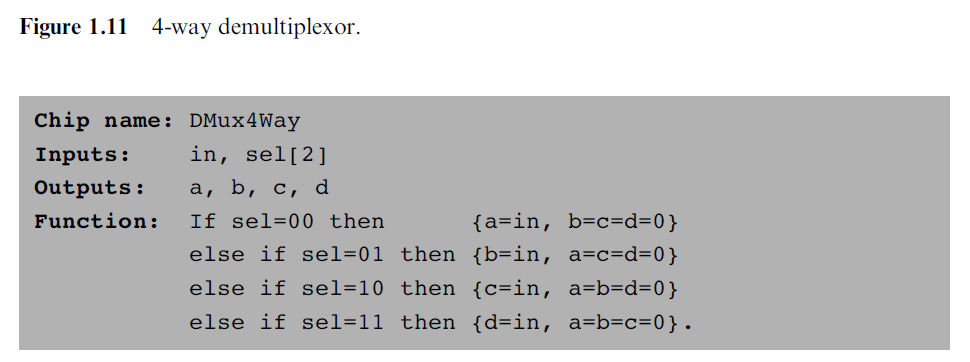# Possible error in 2nd ed spec of multiway demux (p. 24)ClassicListThreaded2 messagesThere may be an error with the way the multiway demux is specified in page 24 (section 1.4) of the second edition of the book. The function reads: ```Input: in, sel Output: out Function: if (sel==00) then {a,b,c,d} = {1,0,0,0}, else if (sel==01) then {a,b,c,d} = {0,1,0,0}, else if (sel==10) then {a,b,c,d} = {0,0,1,0}, else if (sel==11) then {a,b,c,d} = {0,0,0,1} ``` But this means that no matter what the input in is, the output on one of the lines would be 1. I think the function should instead read: ```Input: in, sel Output: out Function: if (sel==00) then {a,b,c,d} = {in,0,0,0}, else if (sel==01) then {a,b,c,d} = {0,in,0,0}, else if (sel==10) then {a,b,c,d} = {0,0,in,0}, else if (sel==11) then {a,b,c,d} = {0,0,0,in} ``` This way, if the output on one of the lines will be match the input, which could be either 0 or 1. I also notice this is more consistent with what's in the first edition.
 I believe you are correct.  The spec certainly doesn't agree with the truth table at the top of the page. The corresponding specification from the 1st edition is:The Dmux8way spec also has the same error.